## Thursday, October 30, 2008

### K-Center and Affinity Propagation Clustering

Recent release of VisuMap implements a new clustering algorithm called Affinity Propagation (AP). AP provides similar service as the metric sampling (MS) algorithm that has been available in VisuMap for a while. In this blog I will compare AP with MS from practical point of view with concrete samples.

First, a few words about the implementations of these algorithms. MS is basically a variation of the k-mean algorithm that uses the expectation maximization (EM) method to solve the k-medoid problem. Such algorithms are sometimes called k-center or k-medoid algorithm. Simple direct implementation of k-medoid algorithm often just find bad solutions (i.e. local minimums). People normally has to repeat the algorithm many many times to find an acceptable solution. The MS algorithm implemented in VisuMap uses a stochastic mechanism to avoid or reduce such local minimum problem.

AP uses message passing mechanism to find representative exemplars within dataset with a similarity structure. AP is basically a deterministic algorithm, it does not require random initialization or randomness during the optimization process. However, AP algorithm often suffers from the oscillation problem in the way that the algorithm wanders around several local minimums for quite long time. To reduce the oscillation, we can use a large dumping factor as suggested in the original paper of AP. But a more efficient way seems to be using small randomness when assigning preferences to the data points. By default, the implementation of AP in VisuMap enables this randomness.

Thus, both MS and AP in VisuMap are of stochasitc nature. I have applied the two algorithms each 500 times on the dataset SP500 (each data point contains the weekly price history of a stock in a year) and got all together 1000 different clustering solutions (each solution is a selection of 25 exemplar points). Using the t-SNE algorithm I created the map of these 1000 solutions as shown in the following picture: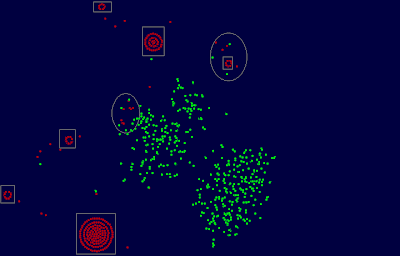In above map each red/green dot represents a solution found by AP/MS algorithm respectively. As a special characteristic of t-SNE algorithm, identical solutions will be arranged as one more circles. That means, dots in a rectangle in above map represent acutally identical solutions.

We notice first that the two algorithms found very different solutions as red and green dots are clearly separated from each other. There are only two small regions (marked by ovals) where the two algorithms found similar solutions. Secondly, we notice that MS found a large number of different solutions. AP found just few different solutions. This means that the AP optimization process often converged to common solutions.

The following table shows the statistics about the solutions found by AP and MS:

 MetricSampling AffinityPropagation Min Error 0.4972 0.4901 Max Error 0.5058 0.5035 Average Error 0.5004 0.4960 Process Timein Seconds 5 4

In the above table the error of a solution is the average distance of the data points to their cluster center (i.e. exemplars). The average error is calculated over the 500 solutions found by each of the two algorithms. We can see that AP has found, on the average, better solutions than MS, but the improvement is only about 0.4%. So, it is fair to say that these two algorithms performed similarly with respect to this dataset.

The situation changed a lot when I applied the two algorithms to a different dataset, namely the dataset PharMine171 discussed in previous blog entry. This dataset contains about 1000 binary 171-dimensional vectors , whereas the SP500 dataset contains 500 40-dimensional vectors with real values. The following is the map of the clustering solutions and the corresponding statistics.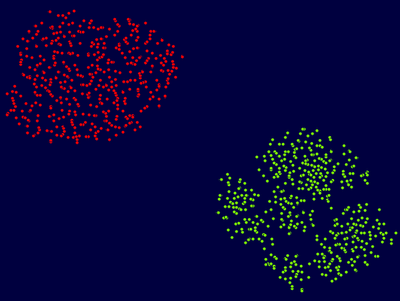MetricSampling AffinityPropagation Min Error 2.1775 2.1101 Max Error 2.2321 2.1686 Average Error 2.1972 2.1603 Process Timein Seconds 6 30

From above map we can see that AP and MS still produced different set of solutions. AP also produced more different solutions than with the previous dataset. This means that, with respect to this dataset, AP is more sensitive to small variations/noise in the input data. This phenomenon might be explained as following: Because this dataset contains binary vectors, many similarities between data points might have exact equal values. Those equivalence of similarity will be broken different by small variations in the input data. Since AP indeed uses operation like max() to distinguish even infinitesimal differences, small variations may lead to significantly different solutions.

We also notice that AP is substantially slower than MS. For larger datasets, the performance difference will be even larger. For instance, for a dataset with about 4000 data points, MS is more than 100 times faster than AP. It should be pointed that both implementations of AP and MS uses the full similarity matrix. It remains to see how much speedup can be achieved by using sparse matrix.

More interestingly we see that the solutions found by AP is about 3.7% better than those found by MS; and even the worst solution found by AP is better than the best solution found by MS.

Now, one would ask the question: Does AP always find better solutions than MS? Unfortunately, this is not the case. The following picture shows the 3D presentation of the spheroid dataset that contains 1825 data points forming together a spheroid: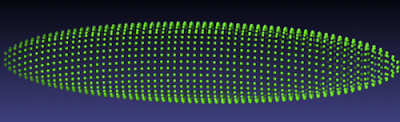As be shown in the following test statistics, solutions found by MS is about 3.7% better than those found by AP:

 MetricSampling AffinityPropagation Min Error 5.9029 5.9029 Max Error 6.4034 6.6483 Average Error 6.0948 6.3199 Process Timein Seconds 9 284

Conclusions:
I believe AP is a useful and promising addition to the arsenal of clustering algorithms. Not only that AP can find better solutions, it also finds different solutions as existing algorithms. As we know that there are many different clustering problems. AP doesn't seem to be designed to directly attack the k-medoid problem, but nevertheless it is competitive to those algorithms directly targeting the k-medoid problem. This indicates that AP may have potential to attack a much larger class of clustering problems.

## Tuesday, October 21, 2008

### Clustering clusters

Many clustering algorithms, like k-mean algorithm, are of stochastic nature in the sense that they produce different partitions (of a data set) depending on the random initialization. In this blog I am going to use the mapping service provided by VisuMap to investigate the partitions generated by a particular clustering algorithm. In other word, I want to visualize the clusters among partitions generated by a clustering algorithm.

The clustering algorithm I am going to look at is the metrical sampling (MS) clustering algorithm which is basically a k-mean algorithm with some randomization extension. For a given set of data points with a distance matrix, MS algorithm tries to find a set of sample points, so that minimizes the average error, that is average distance of the data points to their nearest sample point (also called exemplar). Thus, MS algorithm is one of many available algorithms to solve the so called k-medoid clustering problem (which is an NP complete problem according to certain literature).

For a dataset of N data points, MS algorithm produces a N dimensional binary vectors. Each component is a binary value that decides whether a data point is selected as a sample point. So, when we run the MS algorithm many times with different random initializations, we will get a list of N dimensional binary vectors. I am interested to see clusters or patterns among those binary vectors. For this study, I picked up the dataset PharMine171 that contains 1025 fingerprints of 171 pharmacologically interesting compounds. I have run MS algorithm on the dataset for 1000 times. The following is a map of the 1000 partitions ( produced with the t-SNE mapping algorithm):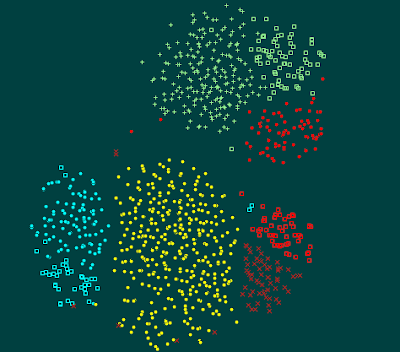In above map, each spot represents a partition generated by one run of MS algorithm. Closely located partitions means they share more sample points. The coloring is done manually to highlight the clusters. We can easily see that there are 8 or 9 clusters among the 1000 partitions.

Now, one question that raises naturally is: Do the partitions in one cluster have similar qualities (i.e. average error)? In order to answer this questions I opened a bar view window that displays the average error of each partition in an ordered bar view as follows: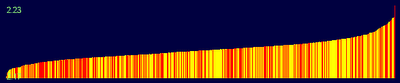In above picture, each bar (in yellow or red color) represents a partition. The height of the bar indicates their average error. The red bars represent those partitions selected manually on the partition maps. So, when we select a cluster in the partition map, we can easily see the corresponding distribution of average error of that clusters. The following two pictures show two such distributions: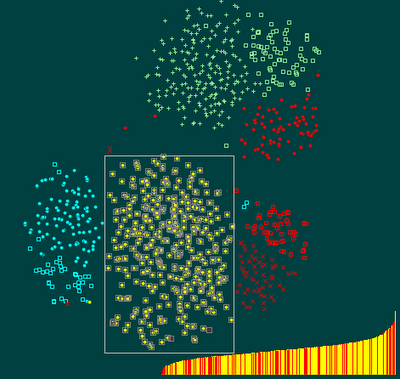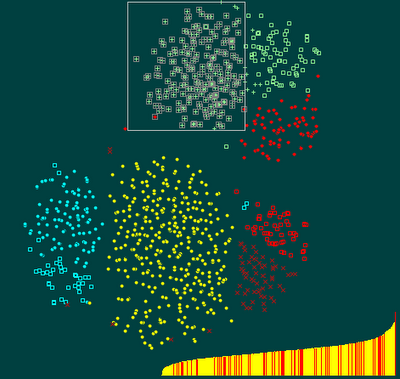We notice that the average error in a cluster is spread all over the whole error range (from very bad to very good). This means, similar partitions can have very different qualities. Thus, the answer to above questions is negative.

More in general, I suppose this study indicates that any algorithm trying to find good solutions for the k-medoid problem need a mechanism to allow global variations in the searching space. The MS algorithm implemented in VisuMap does this through controlled randomness. Another interesting algorithm, named affinity propagation seems to do that with help of 2N2 addition system states (called messages). Affinity propagation is also supported in VisuMap. I will do some comparison between MS and AP in another blog.